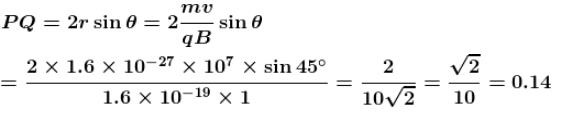Courses

# Magnetic Effect Of Current NAT Level - 1

## 10 Questions MCQ Test Basic Physics for IIT JAM | Magnetic Effect Of Current NAT Level - 1

Description
This mock test of Magnetic Effect Of Current NAT Level - 1 for Physics helps you for every Physics entrance exam. This contains 10 Multiple Choice Questions for Physics Magnetic Effect Of Current NAT Level - 1 (mcq) to study with solutions a complete question bank. The solved questions answers in this Magnetic Effect Of Current NAT Level - 1 quiz give you a good mix of easy questions and tough questions. Physics students definitely take this Magnetic Effect Of Current NAT Level - 1 exercise for a better result in the exam. You can find other Magnetic Effect Of Current NAT Level - 1 extra questions, long questions & short questions for Physics on EduRev as well by searching above.
*Answer can only contain numeric values
QUESTION: 1

### A conductor in the form of a right angle ABC, with AB = 3 cm and BC = 4cm. Carries a current of 10A. There is a uniform magnetic field of 5T perpendicular to the plane of the conductor. The force on the conductor will be (in  Newton)

Solution: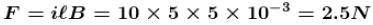*Answer can only contain numeric values
QUESTION: 2

### A short bar magnet of magnetic moment 0.4 J T–1  is place in a uniform magnetic field of 0.16 T. The magnetic field of 0.16 T. The magnetic is stable equilibrium when the potential energy (in Joules)  is.

Solution:

For stable equilibrium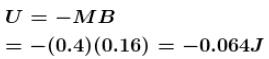*Answer can only contain numeric values
QUESTION: 3

### A beam of protons enters a uniform magnetic field of 0.3T  with velocity of 4 × 105 m/s  in a direction making an angle of 60° with the direction of magnetic field, the pitch of the helix (in metres) will be :

Solution: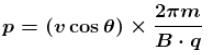*Answer can only contain numeric values
QUESTION: 4

The magnetic flux density at a point distant d from a long straight current carrying conductor is B, then its value at distance d/2 will be αB. Find the value of α.

Solution: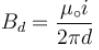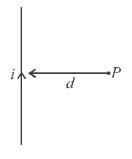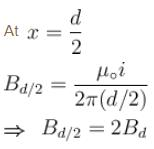*Answer can only contain numeric values
QUESTION: 5

A beam of protons enters a uniform magnetic field of 0.3T with velocity of 4 × 105 m/s  in a direction making an angle of 60° with the direction of magnetic field, the radius (in metres) of path of the particle will be :

Solution: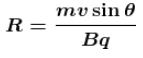*Answer can only contain numeric values
QUESTION: 6

A particle of mass 0.6 g and having charge of 25 nC is moving horizontally with a uniform velocity 1.2 × 104 ms–1  in a uniform magnetic field, then the value of magnetic induction (in Tesla) is. (Take  g = 10ms-2)

Solution:

m = 0.6 gm,   q = 25 nC,   v = 1.2 × 10–4 ms–1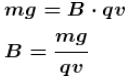*Answer can only contain numeric values
QUESTION: 7

A particle having a charge of 10.0µC and mass  moves in circle of radius 10cm  under the influence of a magnetic field of induction 1T. When the particle is at a point P, a uniform electric field is switched on so that the particle starts moving along the tangent with a uniform velocity. The electric field (in  V/m) is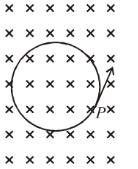Solution: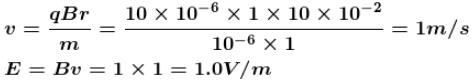*Answer can only contain numeric values
QUESTION: 8

0.5 m  long straight wire in which a current of 1.2 A  is flowing is kept a right angles to a uniform magnetic field of 2.0 tesla. The force (in Newton) acting on the wire will be

Solution: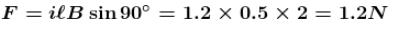*Answer can only contain numeric values
QUESTION: 9

The ratio of magnetic inductions at the centre of a circular coil of radius a  and on its axis at a distance equal to its radius, will be :

Solution: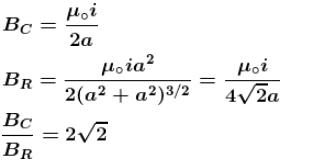*Answer can only contain numeric values
QUESTION: 10

A particle of mass 1.6 × 10–27 kg  and charge 1.6 × 10–19 coulomb  enters a uniform magnetic field of Tesla as shown in the figure. The speed of the particle is 10 m/s. The distance PQ (in metres) will be :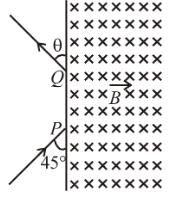Solution: